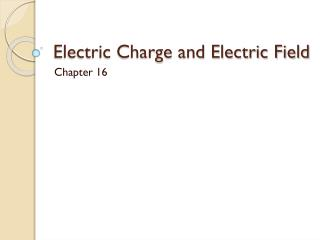DownloadDownload PresentationElectric Charge and Electric Field

Electric Charge and Electric Field

Download PresentationElectric Charge and Electric Field

- - - - - - - - - - - - - - - - - - - - - - - - - - - E N D - - - - - - - - - - - - - - - - - - - - - - - - - - -
Presentation Transcript

1. Electric Charge and Electric Field Chapter 16

2. Electric Charge • Charges • Electrons • Protons • Law of Conservation of Charge • Electric Charge is Quantized • What does it mean to be quantized?

3. Methods of Charging • Insulators vs. Conductors • Types of Charging • Friction • Contact/Conduction • Induction • Polarization?

4. Summarizer Questions • Metals such as copper and silver can become charged by induction, while plastic materials cannot. Why does this happen? • Because of higher moisture content, air is a better conductor of charge in the summer than in the winter. Would you expect the shocks from static electricity to be more severe in summer or in winter? Explain your answer.

5. Electric Force • Opposites Charges Attract • Like Charges Repel • Symbol – F • Units – Newtons • Equation • Coulomb’s Constant vs. Permittivity of Free Space

6. Electric Force Problem A small cork with an excess charge of +6 μCisplaced 0.12 m from another cork, which carries a charge of -4.3 μC. a) What is the magnitude of the electric force between the corks? b) How many excess electrons are on the negative cork?

7. Electric Force Problem • Three point charges lie along the x-axis at x = 0 cm, x = 3.0 cm, and x = 5.0 cm. Calculate the magnitude and direction of the electric force on the middle charge when the charges are +6 μC, +1.5 μC, and -2.0 μC respectively.

8. Summarizer Questions • What are some of the similarities and differences between the electric force and the gravitational force? • The electric force is significantly stronger than the gravitational force. However, although we are attracted to Earth by gravity, we do not usually feel the effects of the electric force? Explain.

9. Electric Field • Permeates space around a charge • Causes an electric force • Depends on strength and distance from charge • Symbol – E • Units – N/C • Equations

10. Electric Field Lines • Point away from positive • Point toward negative • Number of lines proportional to strength • Cannot cross • Zero inside conductor • Accumulates on outside surface of conductor

11. Electric Field Problem • Find the electric field at a point midway between two charges of +40 nC and -60 nC separated by a distance of 30 cm.

12. Summarizer Questions • Explain why you're more likely to get a shock from static electricity by touching a metal object with your finger instead of with your entire hand.